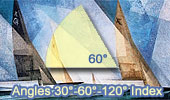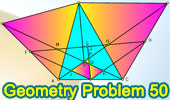Angles 30, 60, 90, 120 Theorems and Problems- Table of Content 2Geometry Problem 619
Triangle Area, 30 Degrees, Incircle, Incenter, Tangent.

Geometry Problem 618
Triangle Area, 60 Degrees, Incircle, Incenter, Tangent.

Proposed Problem 405.
Quadrilateral, 60, 75, and 135 degrees, Midpoint.

Proposed Problem 368.
Triangle, 120 degrees, Angle bisectors, Perpendicular.

Proposed Problem 365.
Circular Sector of 60 degrees, Midpoints, Perpendicular, Congruence.

Proposed Problem 304.
Triangle, Angle bisector of 120 degrees.

Proposed Problem 302.
Triangle, Angle bisector of 60 degrees.

60 and 120 degrees

Proposed Problem 281.
Triangles, Angles, Isosceles, Equilateral.
60 degrees angle.

Proposed Problem 276.
Square, 90 degree Arcs, 60 degrees angle, Circle, Radius.

Proposed Problem 274.
Isosceles Triangle, 80-80-20, Angles.
60 degrees angle.

Proposed Problem 262.
Regular Hexagon inscribed in a circle, sum of distances, 60 degrees angle.

Proposed Problem 260.
Equilateral Triangle, Incircle, Tangency Points, Vertices, Distances, Squares, 60 degrees angle.

Proposed Problem 259.
Equilateral Triangle, Incircle, Tangency Points, Side, Distances, Squares, 60 degrees angle.

Proposed Problem 258.
Equilateral Triangle, Circumcircle, Point, Vertices, Side, Distances, Squares, 60 degrees angle.

Proposed Problem 257.
Equilateral Triangle, Circumcircle, Point, Vertices, Side, Distances, Squares, 60 degrees angle.

Proposed Problem 256.
Equilateral Triangle, Circumcircle, Point, Vertices, Distances, 60 degrees angle.

Proposed Problem 248.
Napoleon's Theorem III. Inner and outer Napoleon triangles, Area, 60 degrees angle.

Proposed Problem 247.
Napoleon's Theorem II. Internal Equilateral triangles. Inner Napoleon triangle, 60 degrees angle.

Proposed Problem 246.
Napoleon's Theorem I. External Equilateral triangles, 60 degrees angle.

Proposed Problem 245. Parallelogram with Equilateral triangles

Proposed Problem 243. Triangle with Equilateral triangles, Parallelogram.

Proposed Problem 242. Triangle with Equilateral triangles, Parallelogram, 60 degrees angle.

Proposed Problem 241. Triangle with Equilateral triangles, Congruence.

Proposed Problem 240. Triangle with Equilateral triangles, Parallelogram, 60 degrees angle.

Proposed Problem 222. Viviani's theorem, Equilateral triangle, Exterior point, 60 degrees angle, Distances.

Proposed Problem 221. Viviani's theorem, Equilateral triangle, Interior point, Distances, 60 degrees angle.

Proposed Problem 212. 120 Degree Triangle, Equilateral triangles, Areas.

Proposed Problem 211. 60 Degree Triangle, Equilateral triangles, Areas.

Proposed Problem 210. Triangle, Angles, Auxiliary Lines, 60 degrees angle.

Proposed Problem 199. Triangle, Quadrilateral, Angles.

Proposed Problem 185. Trapezoid, Triangles and Angles, 60 degrees angle.

Proposed Problem 184. Triangle and Angles, 60 degrees angle.

Proposed Problem 182. Overlapping Circles, Find an angle, 60 degrees angle.

Proposed Problem 132. Triangle, 60 degrees, Orthocenter, Congruence, Midpoint.

Euclid's Elements, Book XIII, Proposition 10 One page visual illustration, 60 degrees angle.

Geometry of Circles "Sesame Street" by Philip Glass, 1979, 60 degrees angle.

Proposed Problem 50. Triangle with Equilateral triangles, 60 degrees angle.
Seventeen conclusions.

Proposed Problem 44. Angles and triangles, 60 degrees angle.

Proposed Problem 43. Angles and triangles.

Proposed Problem 42. Angles and triangles, 60 degrees angle.

Animated illustration of the celebrated Morley's angle trisector theorem, 60 degrees angle, equilateral triangle.

Morley's Triangle & Center: Trisection of angles.Problem 50. Triangle with Equilateral triangles, 60 degrees angle.

Proposed Problem 40.
Triangle, Incenter, Excenter, Angles 80, 40, Distances. Angle bisector,
60 degrees angle.